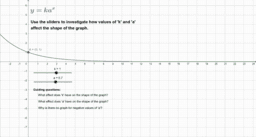•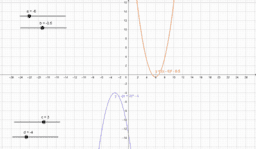Activity

•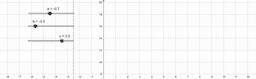Activity

•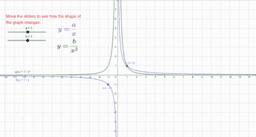Exponential graphs

Activity

•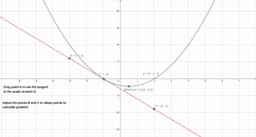Activity

•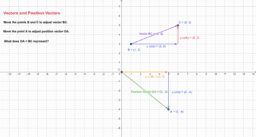Vectors and Position Vectors

Activity

•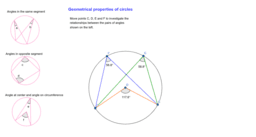Geometrical properties of circles

Activity

•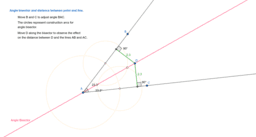Angle Bisector and Equidistance

Activity

•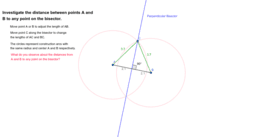Perpendicular Bisector and Equidistance

Activity

•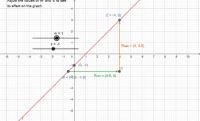Equation of straight line

Activity

•